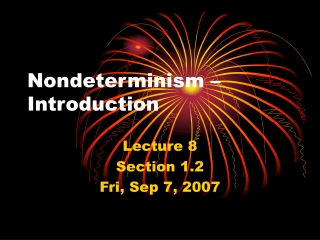# Nondeterminism – Introduction - PowerPoint PPT PresentationDownload PresentationNondeterminism – Introduction

Nondeterminism – Introduction
Download Presentation## Nondeterminism – Introduction

- - - - - - - - - - - - - - - - - - - - - - - - - - - E N D - - - - - - - - - - - - - - - - - - - - - - - - - - -
##### Presentation Transcript

1. Nondeterminism – Introduction Lecture 8 Section 1.2 Fri, Sep 7, 2007

2. Determinism • A finite automaton is deterministic because every move is forced. • That is,  is a function. • For every pair (q, x) Q, there is exactly oneqQ such that (q, x) = q.

3. Nondeterminism • To make a nondeterministicfinite automaton, we relax the requirement that the image of (q, x) be unique. • (q, x) may have no image, one image, or more than one image. • We also allow  moves.

4. Terminology • Deterministic finite automata are called DFAs. • Nondeterministic finite automata are called NFAs.

5. The Language of an NFA • For a given input, an NFA may admit a multitude of computations. • If at least one computation ends in an accept state, then the input is accepted.

6. Concatenation • Let A = {x | x contains an even number of a’s}. • Let B = {x | x contains an even number of b’s}. • Design NFAs that accept • AB • A B • A*

7. Definition of an NFA • To define an NFA, we change the definition of the transition function .

8. Relations and Functions • Given a relation from a set A to a set B, we may derive • A function from A to (B), or • A function from (A) to (B).

9. Example • Let A = {2, 3, 4, 5}. • Let B = {6, 7, 8, 9}. • Let r be the relation defined by (a, b) r if a divides b. • Then r = {(2, 6), (2, 8), (3, 6), (3, 9), (4, 8)}.

10. Example • Define f : A(B) by f(a) = {bB | a divides b}. • Then • f(2) = {6, 8} • f(3) = {6, 9} • f(4) = {8} • f(5) = 

11. Example • Define g : (A) (B) by g(S) = {bB | a divides b for some aS} • Then, for example, • g({2}) = {6, 8} • g({2, 3}) = {6, 8, 9} • What is g()? g({4, 5})?